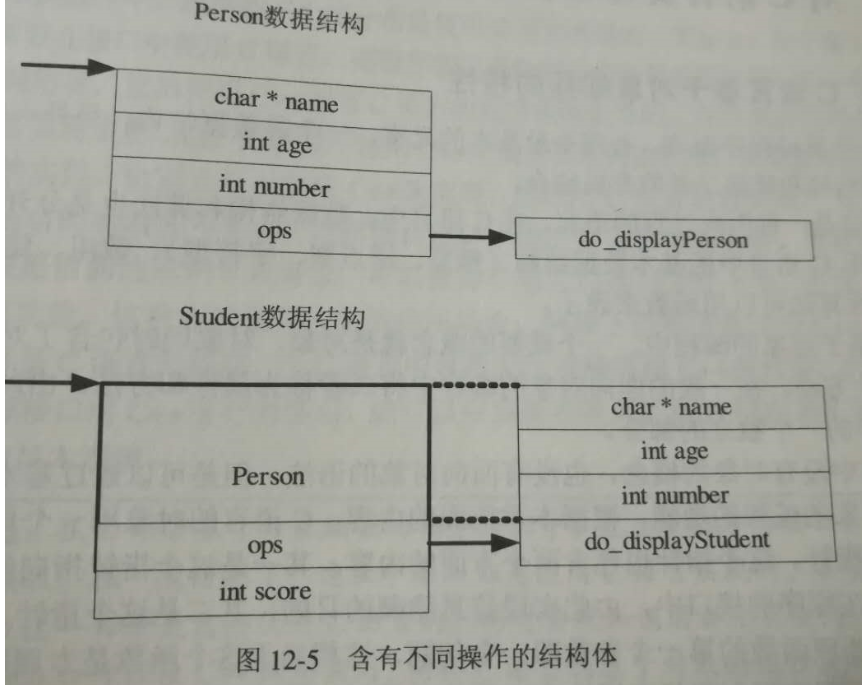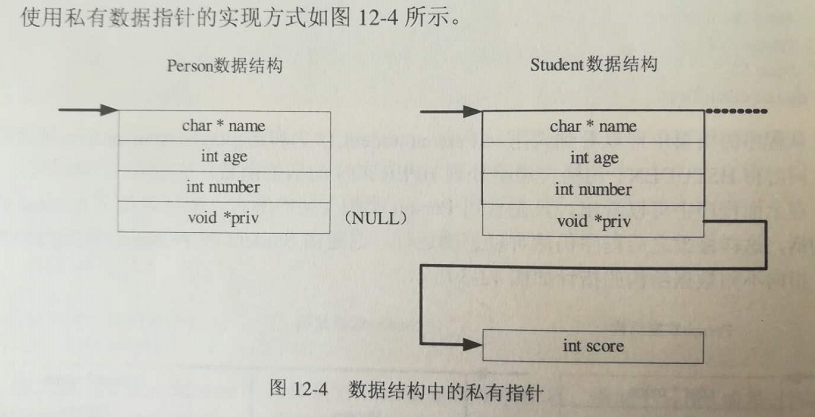• C语言C语言实现面向对象编程之封装代码.，完整的代码，可以在Linux和VS环境运行。【C语言C语言实现面向对象编程之封装代码.rar
• 附件是在VS2012下用C语言实现面向对象的示例代码，并实现设计模式中的工厂模式
• 面向对象编程（OOP）并不是一种特定的语言或者工具，它只是一种设计方法、设计思想。它表现出来的三个最基本的特性就是封装、继承与多态。很多面向对象的编程语言已经包含这三个特性了，例如 Smalltalk、C++、Java。...
• ## C语言实现面向对象

千次阅读 2018-05-10 08:57:46
from : ...C语言不是面向对象语言却也可以用来实现面向对象思想的。有人会觉得用C实现面向对象，充其量是模拟面向对象的语法，而不是像C++那样真的面向对象的。这也算是不太...

from : https://blog.csdn.net/vic_chang/article/details/8457800
C语言是面向过程的语言，可以用来实现面向对象么？答案是肯定的。
面向对象的本质是一种设计思想，与具体的语言没有多大的关系。C语言不是面向对象语言却也可以用来实现面向对象思想的。有人会觉得用C实现面向对象，充其量是模拟面向对象的语法，而不是像C++那样真的面向对象的。这也算是不太正确的看法的。为什么这么说？请先看看C++的类与对象吧class student{  public:     Int getNum()      {}  private:     Int num;};这是一个基本的C++类的声明，当使用这个类定义一个东西的时候，如：student one;编译器也就为one 这个对象只为数据成员分配了空间，即为 int num; 分配了空间，没有为所谓 getNum()方法来分配任何东西的。如果用C来写，就可以这样写：struct student{   Int num;}; Int getNum(){}用C写的这段code与C++写的那段code本质是等效的。不过，有人要说了，C++声明的student类会有构造函数及析构函数，你的C语言就没有。。OK，我们先给上述C++的code加上一个构造函数。class student{  public:    student(int number=0){num = number;}  private:    Int num;};在定义一个student one(1);时， 构造函数就会被调用。构造函数是怎么被调用的呢？？我们好象看不到调用呢？？我们之所以看不到，其实是因为编译器在生成代码的时候，已经在定义后面加入了调用的代码了，这个过程程序员没有参与。也就是等效于： student one; //定义一个对象 student(1); //调用构造函数，由编译器加入的代码。
好了，明白这点，那么，我们的C语言也可以写构造函数了。struct student{   Int num;};
void student(int number, struct student * p){   p->num = number;}
struct student one;   //定义一个结构体对象student(1, &one);   //构造函数
写到现在，用C语言实现面向对象主要有以下几点：1． 在内存分配上，struct 类型与class类型数据是一样的，都只是存储数据的。类的成员函数跟普通函数是一样编译存储的。它所以能跟类关联在一起，实际是由编译器在保证的。用C语言来写的时候，这个关联性由程序员来控制。
2． 对象的构造函数与析构函数的调用，其实是由编译器在做调用。编译器在生成最终的二进制代码的时候，加入了它们的调用代码。类的其他特性也是如此。
3．  类的访问控制private, public, protected这些特性都是由编译器来保证的。最终生成的代码没有这些属性的。4． 用C实现面向对象，其实就是把C++中编译器把所做的部分工作，转为由程序员来做。这样造成的结果无非就是程序员多写一点代码而已。在编译生成的最终汇编代码中，C所实现的面向对象是可以与C++的面向对象保持一致的code.
展开全文• 拉了一个创业团队，准备干一票，去之前也了解了一番，此次将使用C语言来开发，对于毕业之后一直从事C++面向对象思维编码的我来说，虽然不舍，但是仔细想了下，这都不是事，谁说用C语言写不了面向对象？ 　众所周知...
• C语言中还可以实现更深入的面向对象编程多态特性。例如：使用接口(interface)包含多个指向函数的指针，这样就可以实现操作的"多态性"。 在面向对象语言C++的实现上，使用了虚函数的方式，虚函数实质上也是指向虚表...
00. 目录

文章目录
00. 目录01. 概述02. C语言基于对象编程实现部分多态功能03. 总结04. 下载05. 附录

01. 概述
在C语言中还可以实现更深入的面向对象编程多态特性。例如：使用接口(interface)包含多个指向函数的指针，这样就可以实现操作的"多态性"。
在面向对象语言C++的实现上，使用了虚函数的方式，虚函数实质上也是指向虚表(virtual table)的一个函数指针。C++虚表方式的本质和包含的各个函数指针的操作数据结构类似。
02. C语言基于对象编程实现部分多态功能
test.h文件内容如下:
#ifndef __TEST_H__
#define __TEST_H__

#ifdef __cplusplus
//表示是C语言的头文件
extern "C"
{
#endif

typedef void * HPERSON;

//创建对象
HPERSON createPerson(const char *name);

//显示对象
void displayPerson(HPERSON person);

//删除对象
void deletePerson(HPERSON person);

//-----------------STUDENT-----------------
typedef void* HSTUDENT;

//创建对象
HSTUDENT createStudent(const char *name, int age, int id, int score);

//删除对象
void deleteStudent(HSTUDENT student);

#ifdef __cplusplus
}
#endif

#endif /*__TEST_H__*/


test.c具体实现如下:
#define _CRT_SECURE_NO_WARNINGS
#include "test.h"
#include <stdio.h>
#include <stdlib.h>
#include <string.h>

//函数指针
typedef struct _PersonOps
{
void (*display)(HPERSON);
}PersonOps;

//Person表示HPERSON句柄指向的结构体
typedef struct _Person
{
//使用指针
char *name;
int age;
int id;

PersonOps* ops;
}Person;

//显示对象
static void do_displayPerson(HPERSON person)
{
Person* p = person;
if (NULL == p)
{
printf("displayPerson 参数非法\n");
return;
}

printf("Name: %s age: %d id:%d\n", p->name, p->age, p->id);
}

static PersonOps opsPerson = {
do_displayPerson
};

//创建对象
HPERSON createPerson(const char * name)
{
Person *p = NULL;

printf("创建对象\n");

p = malloc(sizeof(Person));
if (NULL == p)
{
printf("分配内存失败\n");
return NULL;
}
memset(p, 0, sizeof(Person));

p->name = malloc(strlen(name) + 1);
if (NULL == p->name)
{
printf("分配内存失败\n");
return NULL;
}

strcpy(p->name, name);
p->age = 0;
p->id = 0;
p->ops = &opsPerson;

return p;
}

//显示对象
void displayPerson(HPERSON person)
{
Person* p = person;

p->ops->display(person);
}

//删除对象
void deletePerson(HPERSON person)
{
Person *p = person;

if (NULL == person)
{
return;
}

if (NULL != p->name)
{
free(p->name);
}

free(person);
}

//---------------------STUDENT--------------------

typedef struct _stu_t {
Person person;
int score;
}Student;

static void do_displayStudent(HSTUDENT student)
{
Student* s = student;

if (NULL == student)
{
return;
}

do_displayPerson(&(s->person));
printf("score: %d\n", s->score);
}

static PersonOps opsStudent = {
do_displayStudent
};

//创建对象
HSTUDENT createStudent(const char* name, int age, int id, int score)
{
Student *s = malloc(sizeof(Student));
if (NULL == s)
{
return NULL;
}
memset(s, 0, sizeof(Student));

s->person.name = malloc(strlen(name) + 1);
if (NULL == s->person.name)
{
return;
}
memset(s->person.name, 0, strlen(name) + 1);

strcpy(s->person.name, name);
s->person.age = age;
s->person.id = id;
s->score = score;

s->person.ops = &opsStudent;
return s;
}

//删除对象
void deleteStudent(HSTUDENT student)
{
Student *s = student;

if (NULL == s)
{
return;
}

if (NULL != s->person.name)
{
free(s->person.name);
}
free(s);

}

main.c实现如下:
#define _CRT_SECURE_NO_WARNINGS
#include<stdio.h>
#include<string.h>
#include<stdlib.h>
#include "test.h"

//主函数
int main()
{

HSTUDENT s = createStudent("李明", 12, 1, 99);

displayPerson(s);
deleteStudent(s);

system("pause");
return 0;
}


测试结果如下：
Name: 李明 age: 12 id:1
score: 99
请按任意键继续. . .


03. 总结
在本例中，含有不同操作的结构体内存格式如下所示PersonOpt数据结构是一个表示"对象"操作的函数表。该程序中它包含了一个操作函数(函数指针)display。在实际应用中，这种函数表数据结构还可能包含更多的数据结构。
总之，利用操作的函数指针列表可以实现部分“多态”功能，其本质是对于不同类型的数据结构调用不同的实现函数。
04. 下载
4.1 代码下载：C语言实现对象编程之多态代码.rar
05. 附录
展开全文多态 C多态 C++
• 没错是C语言面向对象,对于用C出可扩展性强的代码和设计模式应用非常有帮助, 非常有助于嵌入式系统的开发.
• C语言下实现面向对象的三大特性：封装、继承、多态。注意：此文件是vs2010下的工程文件
• 一、类的封装实现：借用高焕堂的宏头文件，类很容易封装为如下的格式 　1、类的定义，其中 CLASS() 是 lw_oopc_kc.h 中定义的...接下来给类 A 的方法实体函数。 void init_A(void *t，int x) { A *cthis = (A
• C语言实现面向对象的方法，面向对象的三个基本特征，对象唯一性，继承性，抽象性。 使用C语言中的结构体和函数指针将数据与操作放在一起，使得一个问题的解决方法封装在结构体中。 其中结构体的嵌套使用实现了继承...
用C语言实现面向对象的方法，面向对象的三个基本特征，对象唯一性，继承性，抽象性。
使用C语言中的结构体和函数指针将数据与操作放在一起，使得一个问题的解决方法封装在结构体中。
其中结构体的嵌套使用实现了继承性。

#include<stdio.h>
#include<stdlib.h>

{
int x;
int y;
int (*imll)(int x, int y);
};

{
int var1;
int var2;
int (*sub)(int x, int y);
};

int sub(int x,int y);
int imll(int x, int y);

int main()
{
a.sub = sub;
a.var3.imll = imll;
a.var1 = 21;
a.var2 = 12;
a.var3.x = 12;
a.var3.y = 13;
a.sub(a.var1, a.var2);
a.var3.imll(a.var3.x,a.var3.y);
return 0;
}

int imll(int x, int y)
{
printf("%d * %d = %d\n",x, y, x*y);
return x*y;
}

{
printf("%d + %d = %d\n", x, y, x+y);
return x+y;
}

int sub(int x, int y)
{
printf("%d - %d = %d\n",x, y, x-y);
return x-y;
}


展开全文函数 语言 继承
• 采用C语言实现的关键是如何运用C语言本身的特性来实现多态、继承面、封装的面向对象的特征,最近给出了例子，大家可以参考使用
• 在基于对象编程实现封装的基础之上，我们接下来实现面向对象编程中的继承部分功能。这种继承特性知识实现部分面向对象编程中继承功能。本博客中我们介绍两种方式的实现继承。第一种是利用数据结构的包含实现继承功能...
00. 目录

文章目录
00. 目录01. 概述02. 利用结构体包含实现继承功能03. 利用私有指针实现继承功能04. 总结05. 附录

01. 概述
面向对象编程具有封装性、继承性、多态性三个基本特性。使用C语言可以实现基于对象的编程。在基于对象编程实现封装的基础之上，我们接下来实现面向对象编程中的继承部分功能。这种继承特性知识实现部分面向对象编程中继承功能。本博客中我们介绍两种方式的实现继承。第一种是利用数据结构的包含实现继承功能，第二种是利用私有指针实现继承功能，它们提供的接口是完全一致的，只是在实现方式上略有不同。
02. 利用结构体包含实现继承功能
在C语言的基于对象编程方面，可以利用结构体包含的扩展实现一定的继承性。下面实现定义一个Student结构，它实现了对Person结构的扩展。
接口文件test.h声明如下
#ifndef __TEST_H__
#define __TEST_H__

#ifdef __cplusplus
//表示是C语言的头文件
extern "C"
{
#endif

typedef void * HPERSON;

//创建对象
HPERSON createPerson(const char *name);

//设置对象
void setPerson(HPERSON person, int age, int id);

//显示对象
void displayPerson(HPERSON person);

//删除对象
void deletePerson(HPERSON person);

#ifdef __cplusplus
}
#endif

//-----------------STUDENT-----------------
typedef void* HSTUDENT;

//创建对象
HSTUDENT createStudent(const char *name);

//设置对象
void setStudent(HSTUDENT student, int age, int id, int score);

//显示对象
void displayStudent(HSTUDENT student);

//删除对象
void deleteStudent(HSTUDENT student);

#endif /*__TEST_H__*/


实现文件test.c如下
#define _CRT_SECURE_NO_WARNINGS
#include "test.h"
#include <stdio.h>
#include <stdlib.h>
#include <string.h>

//Person表示HPERSON句柄指向的结构体
typedef struct _Person
{
//使用指针
char *name;
int age;
int id;
}Person;

//创建对象
HPERSON createPerson(const char * name)
{
Person *p = NULL;

printf("创建对象\n");

p = malloc(sizeof(Person));
if (NULL == p)
{
printf("分配内存失败\n");
return NULL;
}
memset(p, 0, sizeof(Person));

p->name = malloc(strlen(name) + 1);
if (NULL == p->name)
{
printf("分配内存失败\n");
return NULL;
}

strcpy(p->name, name);
p->age = 0;
p->id = 0;

return p;
}

//设置对象
void setPerson(HPERSON person, int age, int id)
{
Person *p = person;

if (NULL != p)
{
p->age = age;
p->id = id;
}
}

//显示对象
void displayPerson(HPERSON person)
{
Person *p = person;
if (NULL == p)
{
printf("displayPerson 参数非法\n");
return;
}

printf("Name: %s age: %d id:%d\n", p->name, p->age, p->id);
}

//删除对象
void deletePerson(HPERSON person)
{
Person *p = person;

if (NULL == person)
{
return;
}

if (NULL != p->name)
{
free(p->name);
}

free(person);
}

//---------------------STUDENT--------------------

typedef struct _stu_t {
Person person;
int score;
}Student;

//创建对象
HSTUDENT createStudent(const char *name)
{
Student *s = malloc(sizeof(Student));
if (NULL == s)
{
return NULL;
}
memset(s, 0, sizeof(Student));

s->person.name = malloc(strlen(name) + 1);
if (NULL == s->person.name)
{
return;
}
memset(s->person.name, 0, strlen(name) + 1);

strcpy(s->person.name, name);
s->score = 0;

return s;
}

//设置对象
void setStudent(HSTUDENT student, int age, int id, int score)
{
Student *s = student;

if (NULL == s)
{
return;
}

setPerson(&(s->person), age, id);

s->score = score;
}

//显示对象
void displayStudent(HSTUDENT student)
{
Student *s = student;

if (NULL == s)
{
return;
}

displayPerson(&(s->person));
printf("Student Score: %d\n", s->score);
}

//删除对象
void deleteStudent(HSTUDENT student)
{
Student *s = student;

if (NULL == s)
{
return;
}

if (NULL != s->person.name)
{
free(s->person.name);
}
free(s);

}

测试文件实现如下
#define _CRT_SECURE_NO_WARNINGS
#include<stdio.h>
#include<string.h>
#include<stdlib.h>
#include "test.h"

int main()
{

HSTUDENT s = createStudent("李明");

setStudent(s, 12, 1, 99);

displayStudent(s);

deleteStudent(s);

system("pause");
return 0;
}


测试结果
Name: 李明 age: 12 id:1
Student Score: 99
请按任意键继续. . .

03. 利用私有指针实现继承功能
在C语言基于对象编程的继承问题上，通常还可以利用私有的指针实现继承。test.h声明如下
#ifndef __TEST_H__
#define __TEST_H__

#ifdef __cplusplus
//表示是C语言的头文件
extern "C"
{
#endif

typedef void * HPERSON;

//创建对象
HPERSON createPerson(const char *name);

//设置对象
void setPerson(HPERSON person, int age, int id);

//显示对象
void displayPerson(HPERSON person);

//删除对象
void deletePerson(HPERSON person);

#ifdef __cplusplus
}
#endif

//-----------------STUDENT-----------------
typedef void* HSTUDENT;

//创建对象
HSTUDENT createStudent(const char *name);

//设置对象
void setStudent(HSTUDENT student, int age, int id, int score);

//显示对象
void displayStudent(HSTUDENT student);

//删除对象
void deleteStudent(HSTUDENT student);

#endif /*__TEST_H__*/


test.c实现如下
#define _CRT_SECURE_NO_WARNINGS
#include "test.h"
#include <stdio.h>
#include <stdlib.h>
#include <string.h>

//Person表示HPERSON句柄指向的结构体
typedef struct _Person
{
//使用指针
char *name;
int age;
int id;

void *priv;
}Person;

//创建对象
HPERSON createPerson(const char * name)
{
Person *p = NULL;

printf("创建对象\n");

p = malloc(sizeof(Person));
if (NULL == p)
{
printf("分配内存失败\n");
return NULL;
}
memset(p, 0, sizeof(Person));

p->name = malloc(strlen(name) + 1);
if (NULL == p->name)
{
printf("分配内存失败\n");
return NULL;
}

strcpy(p->name, name);
p->age = 0;
p->id = 0;

//私有数据初始化
p->priv = NULL;

return p;
}

//设置对象
void setPerson(HPERSON person, int age, int id)
{
Person *p = person;

if (NULL != p)
{
p->age = age;
p->id = id;
}
}

//显示对象
void displayPerson(HPERSON person)
{
Person *p = person;
if (NULL == p)
{
printf("displayPerson 参数非法\n");
return;
}

printf("Name: %s age: %d id:%d\n", p->name, p->age, p->id);
}

//删除对象
void deletePerson(HPERSON person)
{
Person *p = person;

if (NULL == person)
{
return;
}

if (NULL != p->name)
{
free(p->name);
}

free(person);
}

//---------------------STUDENT--------------------

typedef struct _stu_t {
int score;
}StudentPriv;

//创建对象
HSTUDENT createStudent(const char *name)
{
Person *p = NULL;

p = malloc(sizeof(Person));
if (NULL == p)
{
printf("分配内存失败\n");
return NULL;
}
memset(p, 0, sizeof(Person));

p->name = malloc(strlen(name) + 1);
if (NULL == p->name)
{
printf("分配内存失败\n");
return NULL;
}

strcpy(p->name, name);
p->age = 0;
p->id = 0;

//创建Student的私有数据
p->priv = malloc(sizeof(StudentPriv));
if (NULL == p->priv)
{
printf("分配内存失败\n");
return NULL;
}

((StudentPriv*)p->priv)->score = 0;

return p;
}

//设置对象
void setStudent(HSTUDENT student, int age, int id, int score)
{
Person *s = student;

if (NULL == s)
{
return;
}

setPerson(s, age, id);

((StudentPriv*)s->priv)->score = score;
}

//显示对象
void displayStudent(HSTUDENT student)
{
Person *s = student;

if (NULL == s)
{
return;
}

displayPerson(s);
printf("Student Score: %d\n", ((StudentPriv*)s->priv)->score);
}

//删除对象
void deleteStudent(HSTUDENT student)
{
Person *s = student;

if (NULL == s)
{
return;
}

if (NULL != s->name)
{
free(s->name);
}
free(s);

}

测试程序main.c实现如下
#define _CRT_SECURE_NO_WARNINGS
#include<stdio.h>
#include<string.h>
#include<stdlib.h>
#include "test.h"

int main()
{

HSTUDENT s = createStudent("李明");

setStudent(s, 12, 1, 99);

displayStudent(s);

deleteStudent(s);

system("pause");
return 0;
}


测试结果
Name: 李明 age: 12 id:1
Student Score: 99
请按任意键继续. . .


04. 总结
C语言可以实现部分继承功能。实现对象的继承利用的是C语言编译后的内存布局。这种继承方式可以实现将扩展对象的句柄传递给基本对象。由于内存布局的特点，C语言基于对象的编程无法无法实现多重继承。
由于程序中需要隐藏细节，这种继承只能在下层的程序中定义，而很难从在调用程序中扩展。因此这种C语言所实现的知识基于对象的编程，而不能实现完全的面向对象编程。
总之，使用C语言进行基于对象编程的继承特性的时候，更多的是在下层提供的接口中，提供多个句柄表示有继承关系的对象，被继承者的处理函数可以处理继承者的句柄。
05. 附录
代码下载：C语言实现对象编程之继承代码.rar
展开全文C继承
• 对于编程而言，重要的是解决问题的方式，而不是语言本身。面向对象与面向过程是解决...C语言常说是面向过程开发的语言，因为其缺少很多对于面向对象特性的支持。但，这并不影响我们采用面向对象的方式，使用C语言编程。
• 00. 目录 文章目录00. 目录01. 前言02. 简单程序示例03. 程序示例优化04. 总结05....01. 前言 面向对象编程（OOP）并不是一种特定的语言或者工具，它只是一种设计方法、设计思想。...C语言不是面向对象的语言，因此使
• 可喜的是，C语言通过适当组织处理，也可以类似于C++一样面向对象编程。下面就来介绍这种组织处理的过程（受linux C源码的启发）。 1.事物归类 面向对象，就是将研究或操作的硬件或变量（所谓对象）根据其属性及操作...
• 我们经常说C语言是面向过程的，而C＋＋是面向对象的，然而何为面向对象，什么又是面向过程呢？不管怎么样，我们最原始的目标只有一个就是实现我们所需要的功能，从这一点说它们是殊途同归的。过程与对象只是侧重点...
• 最近百度面试过程中有同学被问到这样一个问题：如何用C语言实现面向对象？我们都知道面向对象的三大基本特征：封装、继承和多态，C++语言和编译器都对这些特征有着强有力的支持，但是对于C这样的函数式语言，如何...设计 多态 继承
•   As we all konw，Linux操作系统大部分都是用C语言写的，而在编写linux中，大佬们优美的应用C语言写出了OO（面向对象）的操作系统。   关于Linux内核中利用的OO思想的具体体现，网上有很多好的文章，我在这就不...封装 继承 多态
• C语言是一种结构化的编程语言，以...C语言本身对面向对象的支持很弱，但可以通过一些技巧来实现，从面向对象的思想上找一些对应的实现方法。 面向对象C语言： 类的实现 ———— 结构体 封装性  ————继承 多态 对象...

# c语言写面向对象c语言 订阅